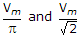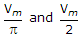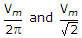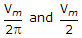# Electronics and Communication Engineering - Power Electronics

### Exercise :: Power Electronics - Section 6

16.

Two thyristors of the same rating and specifications

 A. will have equal on state voltage drops B. will have equal forward blocking voltage for the same current C. will have different on state voltage drops D. may have equal or different on state voltage drops and equal or different forward blocking voltage's for the same current

Explanation:

No answer description available for this question. Let us discuss.

17.

A 3 phase induction motor is controlled by voltage and frequency control such that the ratio V/f is constant. At 50 Hz, the starting torque is 100 N-m. At 25 Hz, the starting torque

 A. will be less than 100 N-m B. will be more than 100 N-m C. may be more or less than 100 N-m depending on motor parameters D. very small

Explanation:

No answer description available for this question. Let us discuss.

18.

In a three phase to single phase full wave bridge cycloconverter, the number of thyristors is

 A. 36 B. 18 C. 12 D. 8

Explanation:

No answer description available for this question. Let us discuss.

19.

A single phase half wave converter is feeding-a resistor load. The input voltage is v = Vm sin ωt. If firing angle is zero, the output dc voltage Vdc and output rms voltage Vrms respectively

 A.B.C.D.Explanation:

No answer description available for this question. Let us discuss.

20.

In a cycloconverter it is possible to vary

 A. only output frequency B. only output voltage C. both output voltage and frequency but not simultaneously D. both output voltage and output frequency simultaneously